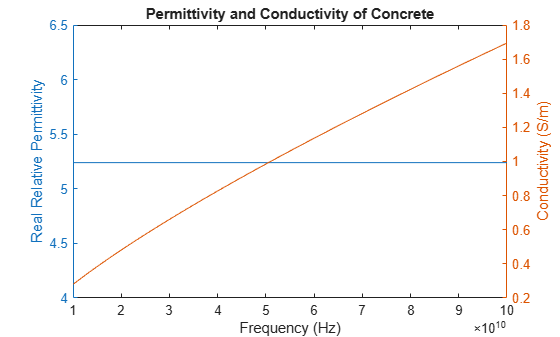# buildingMaterialPermittivity

Permittivity and conductivity of building materials

## Syntax

``[epsilon,sigma,complexepsilon] = buildingMaterialPermittivity(material,fc)``

## Description

example

````[epsilon,sigma,complexepsilon] = buildingMaterialPermittivity(material,fc)` calculates the relative permittivity, conductivity, and complex relative permittivity for the specified material at the specified frequency. The methods and equations modeled in the `buildingMaterialPermittivity` function are presented in Recommendation ITU-R P.2040 .```

## Examples

collapse all

Calculate relative permittivity and conductivity at 9 GHz for various building materials as defined by textual classifications in ITU-R P.2040, Table 3.

```material = ["vacuum";"concrete";"brick";"plasterboard";"wood"; ... "glass";"ceiling-board";"chipboard";"floorboard";"metal"]; fc = repmat(9e9,size(material)); % Frequency in Hz [permittivity,conductivity] = ... arrayfun(@(x,y)buildingMaterialPermittivity(x,y),material,fc);```

Display the results in a table.

```varNames = ["Material";"Permittivity";"Conductivity"]; table(material,permittivity,conductivity,'VariableNames',varNames)```
```ans=10×3 table Material Permittivity Conductivity _______________ ____________ ____________ "vacuum" 1 0 "concrete" 5.31 0.19305 "brick" 3.75 0.038 "plasterboard" 2.94 0.054914 "wood" 1.99 0.049528 "glass" 6.27 0.059075 "ceiling-board" 1.5 0.0064437 "chipboard" 2.58 0.12044 "floorboard" 3.66 0.085726 "metal" 1 1e+07 ```

Calculate the relative permittivity and conductivity for concrete at frequencies specified.

```fc = ((1:1:10)*10e9); % Frequency in Hz [permittivity,conductivity] = ... arrayfun(@(y)buildingMaterialPermittivity("concrete",y),fc);```

Plot the relative permittivity and conductivity of concrete across the range of frequencies.

```figure yyaxis left plot(fc,permittivity) ylabel('Relative Permittivity') yyaxis right plot(fc,conductivity) ylabel('Conductivity (S/m)') xlabel('Frequency (Hz)') title('Permittivity and Conductivity of Concrete')```## Input Arguments

collapse all

Building material, specified as vector of strings including one or more of these options:

 `"vacuum"` `"glass"` `"very-dry-ground"` `"concrete"` `"ceiling-board"` `"medium-dry-ground"` `"brick"` `"floorboard"` `"wet-ground"` `"plasterboard"` `"chipboard"` `"wood"` `"metal"`

Example: `["vacuum" "brick"]`

Data Types: `char` | `string`

Carrier frequency in Hz, specified as a positive scalar.

### Note

`fc` must be in the range [1e6, 10e6] when the `material` is `"very-dry-ground"`, `"medium-dry-ground"` or `"wet-ground"`.

Data Types: `double`

## Output Arguments

collapse all

Relative permittivity of the building material, returned as a nonnegative scalar or row vector. The output dimension of `epsilon` matches that of the input argument `material`.

Conductivity, in Siemens/m, of the building material, returned as a nonnegative scalar or row vector. The output dimension of `sigma` matches that of the input argument `material`.

Complex relative permittivity of the building material, returned as a complex scalar calculated by

`complexepsilon` = `epsilon` – 1i `sigma` / (2π`fc`ε0),

where ε0 = 8.854187817e-12 Farads/m. ε0 is the electric constant for the permittivity of free space.

The output dimension of `complexepsilon` matches that of the input argument `material`.

 ITU-R P.2040-1. "Effects of Building Materials and Structures on Radiowave Propagation Above 100MHz." International Telecommunications Union - Radiocommunications Sector (ITU-R). July 2015.